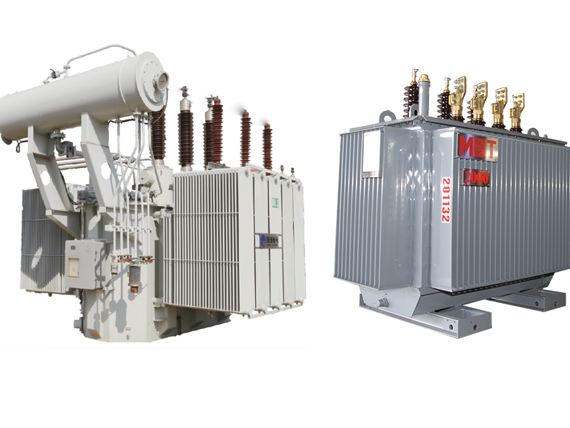# STEP DOWN TRANSFORMER/ MBT TRANSFORMER

A transformer is a kind of electrical equipment that converts high voltage to low voltage or the opposite. Based on the voltage conversion, there are two main types of transformers as Step-up transformer and step-down transformer. This article shows you the knowledge of step-down transformers.

1. What is a Step-Down Transformer?
3. Application

## What is a Step-Down Transformer?

A step-down voltage transformer is a type of transformer that is used to reduce the output voltage. Besides that, it transforms high voltage, low current power into low voltage, high current power. For example, the power supply has a voltage of 22KV, but the factory only requires 0.4KV. Therefore, it is recommended to use a step-down transformer to reduce the voltage from 22KV to 0.4KV.

Due to safety reasons, in many regions, the voltage is reduced to 440v / 230v, so the number of turns on the secondary is less than that once on the primary; Less voltage is generated at the transformer's output (secondary) end.

Regarding the operation voltage, the step-up transformer application can be divided into two groups: HV application (voltages above 1 kV) and LV (voltages up to 1 kV).

A transformer can convert the voltage from a higher primary side voltage to a lower secondary side voltage and also step up the voltage from a lower primary side voltage to a higher secondary side voltage.

For a step-down transformer, the transformer turns ratio (n) is approximately proportional to the voltage ratio:Where VP,S are voltages

NP,S are the turn numbers on the primary (LV) and secondary (HV) sides respectively.

The secondary side of a step-down transformer (LV side) has a smaller number of turns than the primary side (HV side).

That means energy flows from the HV to the LV side and step down the voltage is stepped down from the primary voltage (input voltage) to the secondary voltage (output voltage).

This equation can be rearranged to give the formula for output voltage (i.e. secondary voltage). This is sometimes called the step-down transformer formula:A step-down transformer has the function of providing this low voltage value which is suitable for electronics supply. It converts home voltage (230/120 V) from primary to a low voltage on the secondary side used for electronic supplying.

Transformers with high operating frequency is used (kHz-s) if electronic devices are designed to have higher nominal power. 50/60 Hz nominal frequency would be too large and heavy if the transformers with higher nominal power value. And also, the daily used battery chargers use the step-down transformer in their design.

The transformer that has a smaller number for secondary winding and a larger number of turns in the primary winding is called a step-down transformer. So, as we can see from the previous equation for the relation between the number of turns in winding and voltage, if the number of turns in the secondary is less than the number of turns in primary then the EMF generated in the secondary is less than the primary input.

• Used to provide high currents and low voltages.
•  very efficient and can give the desired output with an efficiency of up to 99 percent.
•  less expensive and more reliable.
• Able to get the desired output voltage easily without loss of much power.

Related Articles:

Step up vs step down transformer

Distribution Transformer

## Application of Step-Down Transformer

The step-down transformers play an important role in a power system. They reduce the voltage level and adapt it to energy consumers.

Other applications include:

• Voltage stabilizers and inverters
• Power transmission lines
• Welding machines

.(+84) 0913.006.538
Skype, Telegram, Wechat ...